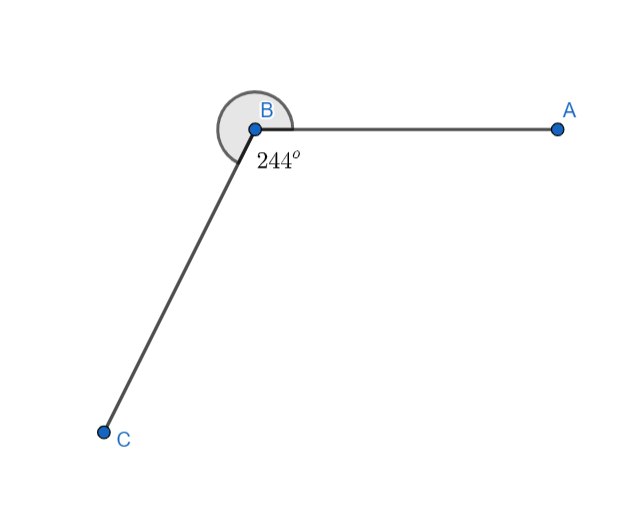Courses
Courses for Kids
Free study material
Free LIVE classes
MoreLIVE
Join Vedantu’s FREE Mastercalss

# Which of the following is a reflex angle?A) ${{180}^{o}}$ B) ${{360}^{o}}$C) ${{204}^{o}}$D) ${{135}^{o}}$Verified
362.4k+ views
Hint: The given question is related to types of angles. Try to recall the various types of angles, their definition, and their examples.

In mathematics, an angle is defined as the measure of inclination of one line (or line segment) on another line (or line segment). Based on the measure of the angle, angles are classified into six types:
A) Acute angle
B) Right angle
C) Obtuse angle
D) Straight angle
E) Reflex angle
F) Complete angle (or Full angle)
Acute angle: Any angle whose measure is more than ${{0}^{o}}$ and less than ${{90}^{o}}$ is called an acute angle.
Right angle: The angle whose measure is exactly ${{90}^{o}}$ is called a right angle.
Obtuse angle: Any angle whose measure is more than ${{90}^{o}}$ and less than ${{180}^{o}}$ is called an obtuse angle.
Straight angle: The angle whose measure is exactly ${{180}^{o}}$ is called a straight angle.
Reflex angle: Any angle whose measure is more than ${{180}^{o}}$ and less than ${{360}^{o}}$ is called a reflex angle.
Complete angle: The angle whose measure is exactly ${{360}^{o}}$ is called a complete angle.
The figure of a reflex angle is shown below:The measure of the angle in the figure is equal to ${{244}^{o}}$ , which lies between ${{180}^{o}}$ and ${{360}^{o}}$ . Hence, it is a reflex angle.
Now, coming to the question, we need to find the reflex angle among the given options. We can see that in the given options, option C. ${{204}^{o}}$ lies between ${{180}^{o}}$ and ${{360}^{o}}$ . Hence, it is a reflex angle.
So, option C is the correct answer.

Note: Students are generally confused between obtuse angles and reflex angles. Obtuse angles are those angles whose measure is more than ${{90}^{o}}$ and less than ${{180}^{o}}$, whereas, reflex angles are those angles whose measure is more than ${{180}^{o}}$ and less than ${{360}^{o}}$. Such confusion should be avoided.
Last updated date: 29th Sep 2023
Total views: 362.4k
Views today: 7.62k